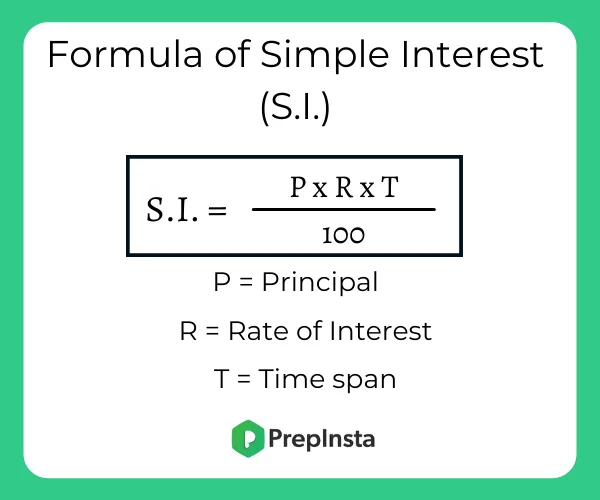# Formulas for Simple Interest Problems

## Formulas to Solve Simple interest Problems in Aptitude

Simple interest is a easy method of calculating the interest charge on a loan. First, it is important to recall the concept of interest and ways to calculate it. This Page from here on contains Formulas and definition of Simple Interest.### Simple Interest Formulas

• Simple Interest is the rate at which we lend or borrow money. In simple terms, when a lender lends money to a borrower, the borrower has to pay an extra amount of money to the lender.  This extra amount of money is called interest. The interest on a sum borrowed for a certain period is called simple interest.• After the calculation for S.I. is done, the principal has to be added to it to get the total amount that the borrower has to give or the lender will collect. This is called total amount and its formula is given as:

### Notations in S.I. Formula:

 S.I Simple Interest P Principal Amount A Total Amount R Rate of Interest T Time (in Years)

Using the above notations, the formula for S.I. becomes,

S.I.=$\frac{P * R * T}{100}$

This formula can be used to find the missing parameters while calculating the interest or total amount. Thus, the reduced forms of this formula are:

To calculate the Interest, the formula becomes:

• I = PTR/100

To calculate the Principal Amount, the formula is:

• P = (I × 100) / RT

To find the rate of interest, the formula will be:

• R = (I × 100)/ PT
• R (in decimal ) = I/PT

Thus, the rate of interest in percent is given by:

• R = R * 100

To get the time, formula is:

• T = (I × 100) / PR

### Related Banners

Get PrepInsta Prime & get Access to all 200+ courses offered by PrepInsta in One Subscription

### Formulas based Questions on Simple Interest

Example 1.  Find the simple interest on Rs. 65,000 at 6(2/3)% per annum for a period of 9 months?

Options
(A) 3520
(B) 3250
(C) 2350
(D) 5320

Solution      Principle = 65,000

Rate = 6$\frac{2}{3} \$

Time = 9 months

Principle = 65,000

Rate = $\frac{20}{3} \$

Time =$\frac{3}{4} \$

S.I = $\frac{P*R*T}{100} \$

= Rs. [65000×(20/3)×(3/4)×(1/100)]

= 3250

Correct Option(B)

Example 2. What sum of money will amount to Rs. 520 in 5 years and Rs. 568 in 7 years at simple interest?

Options
(A) 350
(B) 400
(C) 550
(D) 500

Solution     Amount in 5 years = Rs 520

Amount in 7 years = Rs 568

2 years  S.I = 568-520 = 48

Simple Interest for 1 years = $\frac{48}{2} \$ = 24

5 years amount = Rs 520

For 1 years = 5*24 = 120

P = A – S.I = 520-120

P = 400

Correct Option(B)

Example 3 : At what rate, percent per annum will a sum of money triple in 13 years approximately.

Options
(A) 2.25%
(B) 8.98%
(C) 15.38%
(D) None

Solution : Let principal = P, Then, S.I.= 2P and Time = 13 years
We know that S.I. = $\frac{PTR}{100}$
Rate = $\frac{100*2P}{P*13}$% = 15.38% per annum.

Correct option : 15.38%

Example 4 : A sum was put at simple interest at a certain rate for 9 years. Had it been put at a 5% higher rate, it would have fetched Rs 540 more. Find the sum.

Options
(A) 5278 rs
(B) 1200 rs
(C) 627 rs
(D) 3200 rs

Solution:
Let sum = P and original rate = R. Then

$[\frac{(P \times (R+5) \times 9)}{100}] – [\frac{(P \times R \times 9)}{100}] = 540$
$9P \times (R+5) – 9PR = 54000$
9PR + 45P – 9PR = 54000
45P = 54000
P = 1200

Correct Option : 3200 Rs

Example 5 : A sum of money at simple interest amounts to Rs. 873 in 6 years and to Rs. 923 in 9 years. The sum is:

Options
(A) 624 rs
(B) 573 rs
(C) 362 rs
(D) none

Solution:  S.I. for 1 year =  Rs. (923 – 873) = Rs. 50.

S.I. for 6 years = Rs.(50 * 6) = Rs. 300.

Principal = Rs. (873 – 300) = Rs. 573

Correct Option: 573 Rs

## Get over 200+ course One Subscription

Courses like AI/ML, Cloud Computing, Ethical Hacking, C, C++, Java, Python, DSA (All Languages), Competitive Coding (All Languages), TCS, Infosys, Wipro, Amazon, DBMS, SQL and others